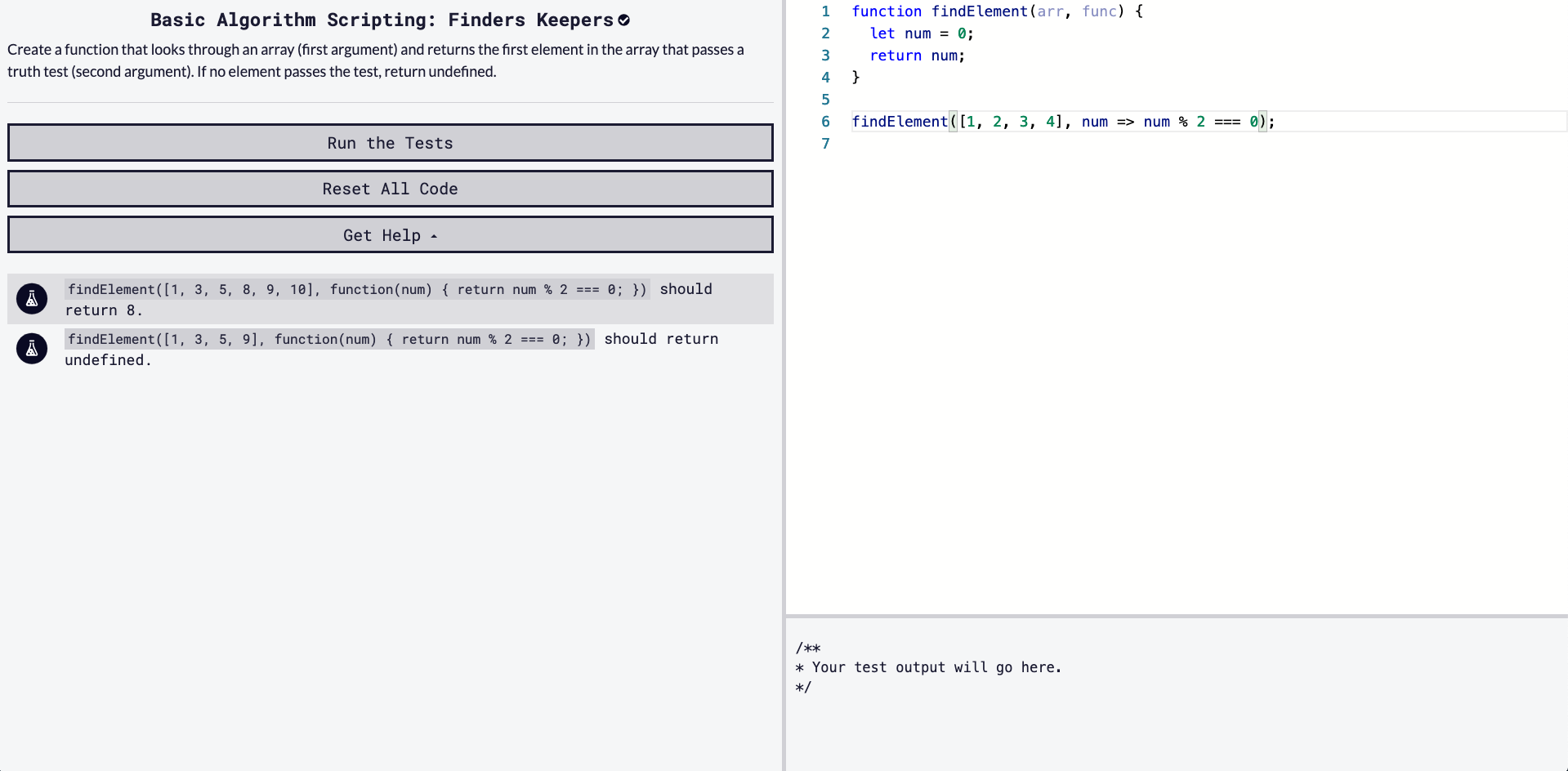## DEV Community 👩‍💻👨‍💻

Viren B

Posted on • Originally published at virenb.cc

# Solving "Finders Keepers" / freeCodeCamp Algorithm Challenges

Post can also be found on my website https://virenb.cc/fcc-009-finders-keepersLet's solve freeCodeCamp's Basic Algorithm Scripting Challenge, "Finders Keepers"

## Our Starter Code (& Tests)

``````function findElement(arr, func) {
let num = 0;
return num;
}

findElement([1, 2, 3, 4], num => num % 2 === 0);

``````
``````// Tests
findElement([1, 3, 5, 8, 9, 10], function(num) { return num % 2 === 0; }) should return 8.
findElement([1, 3, 5, 9], function(num) { return num % 2 === 0; }) should return undefined.
``````

### Our Instructions

Create a function that looks through an array (first argument) and returns the first element in the array that passes a truth test (second argument). If no element passes the test, return undefined.

## Thoughts

• We have two arguments as inputs, one being a `arr` being an array, `func` being a function
• We have to return a number or undefined, based on tests
• Will have to compare the array in `arr` against the constraints in `func`

### Further Thoughts

Reading the instructions again, we will have to check the array against the function provided in `func`, returning the first `num` which meets the constraint. If there are no values in the array which pass the 'test' in `func`, return `undefined`.

So reading this, I figure we should be using some sort of loop on the `arr`, checking each value against the `func` test.

I usually will try to use a more modern method, like `map()`, but I don't think it is the best case since we kind of have to 'break' out of the loop once we meet the first value which is true against the `func` test.

The starter code gives us `let num = 0`. I will change that to `let num;` so it is `undefined` to start with. If no values within the array pass the test, we will still return `num`, giving us `undefined`.

I will opt for a for loop, looping through the array, and will set `num` to the value of the array's index which is `true`, then immediately return `num`;

I want to immediately return the first value because of one of the tests. Looking at the below,

`findElement([1, 3, 5, 8, 9, 10], function(num) { return num % 2 === 0; }) should return 8.`

Both 8 and 10 are divisble by 0, so if we do not break out of the loop, num would eventually be set to 10, overwriting 8. We want 8 though.

Here is a little pseudo pseudocode:

``````function findElement(arr, func) {
create num variable, assign no value yet
for (let i = 0; i < arr's size; i++) {
if (func(arr[i]) is true)
set num equal to arr[i]
return num to stop the loop

return num
}

``````

## Solution

[SPOILER: SOLUTION TO CODE BELOW]

``````function findElement(arr, func) {
let num;
for (let i = 0; i &lt; arr.length; i++) {
if (func(arr[i])) {
num = arr[i];
return num;
}
}
return num;
}
``````

Repeat a String Repeat a String Challenge on fCC

freeCodeCamp

Donate to FCC!

Solution on my GitHub